# ILP Part 34 — Special Ordered Set Type 1 and 2

This is the thirty fourth part of the ILP series. For your convenience you can find other parts in the table of contents in Part 1 – Boolean algebra

In the next part of ILP series we will implement Special ordered sets. These two constraints are pretty simple in their idea, Special ordered set of type 1 (SOS1) is used when we want to constraint that at most one of the variable has non-zero value. Special ordered set of type 2 (SOS2) adds two constraints: at most two variables can be non-zero, and if it is the case, these variables must be consecutive in their ordering. So setis SOS2, whereas set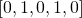is not (since non-zero variables do not stand next to each other).

Let’s begin with SOS1.

# Special ordered set of type 1

We have the following variables: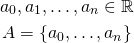For each index we create another binary variable: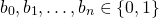We demand that at most one binary is non-zero: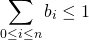And we specify constraint which will allow matching variable to take non-zero value: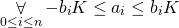Where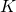is maximum integer value which our solver supports.

How does it work? There can be at most one non-zero binary flag, and only for that flag the respective variable is allowed to take non-zero value (because for all the other flags constraint will have zero on both sides). However, non-zero flag does not require matching variable to be non-zero, but this does not break definition of SOS1.

# Special ordered set of type 2

Now we want to add constraint that at most two variables are non-zero, and if there are two non-zero variables, they are next to each other. Using the same variables as before:We create binary variables in the same way and still require that at most one binary is non-zero: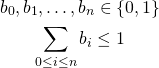This time for each variable we take two binary flags into the constraint: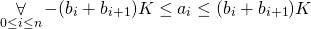How does it work? Let’s assume that all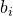are zeroes, this means that we have lots of constraints of the form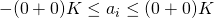which makes sure that all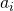are zeroes. However, imagine that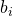is true. This time we have two interesting constraints: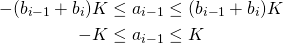and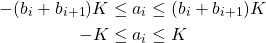So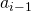andare allowed to be non-zero, so at most two variables can be non-zero.

# Summary

Special ordered sets looks easy but they are very useful. In next part we will see how to approximate any 1D or 2D function using SOS2.